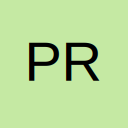# can any one change this code into worksheet event change macro in general format only

• i have applied this macro to add +91- before mobile number of 10 digits

here can any one change this code into worksheet event change macro because

when i type mobile number in a cell +91- should be add automatically

here i know we can add +91- in cell range by changing cell range formating into format cells

in format cells we have apply custom format to add +91- before mobile number

here i want to add +91- before mobile number in general format only

Code
``````Sub Add()
For Each r In Range("A1:A4")
If Not (Left(r.Value, 2) = "+91-") Then
r.Value = "+91-" & r.Value
End If
Next r
End Sub``````
• Can you change this code into worksheet change event

• This should work, adjust the range if required.

Code
``````Private Sub Worksheet_SelectionChange(ByVal Target As Range)
If Intersect(Target, Range("A1:A4")) Is Nothing Then Exit Sub
Const s As String = "+91-"
If Len(Target) = 10 Then Target.Value = s & Target.Value
End Sub``````
• Thank you so much royUK

it's working perfectly

i have applied this code like this it's working thank you so much

Code
``````Private Sub Worksheet_Change(ByVal Target As Range)
On Error Resume Next
If Intersect(Target, Range("A1:A20")) Is Nothing Then Exit Sub
Const s As String = "+91-"
If Len(Target) = 10 Then Target.Value = s & Target.Value
End Sub``````
• why i have added on error resume next

When i have applied this code into worksheet change and i went to sheet and enter a mobile number in a cell

Then i get run time error

• i have applied code in excel 2007 version

I am using excel 2007

## Participate now!

Don’t have an account yet? Register yourself now and be a part of our community!